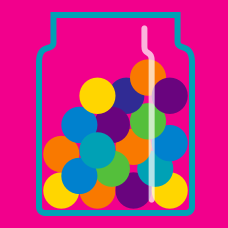Probability

# Bayes' Theorem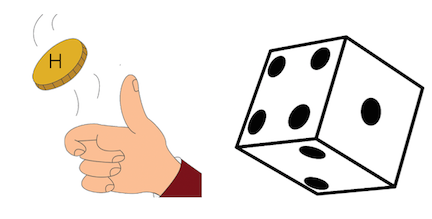You flip a coin and roll a die. Let $H$ be the event you flip a heads and let $F$ be the event that you roll a 4. What is $P\left(H\ | \ F\right)?$

Note: $P\left(H\ | \ F\right)$ denotes the probability of $H$ occurring given that $F$ occurs.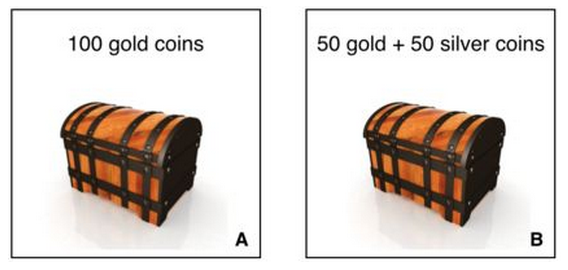You randomly choose a treasure chest to open, and then randomly choose a coin from that treasure chest. If the coin you choose is gold, then what is the probability that you chose chest A?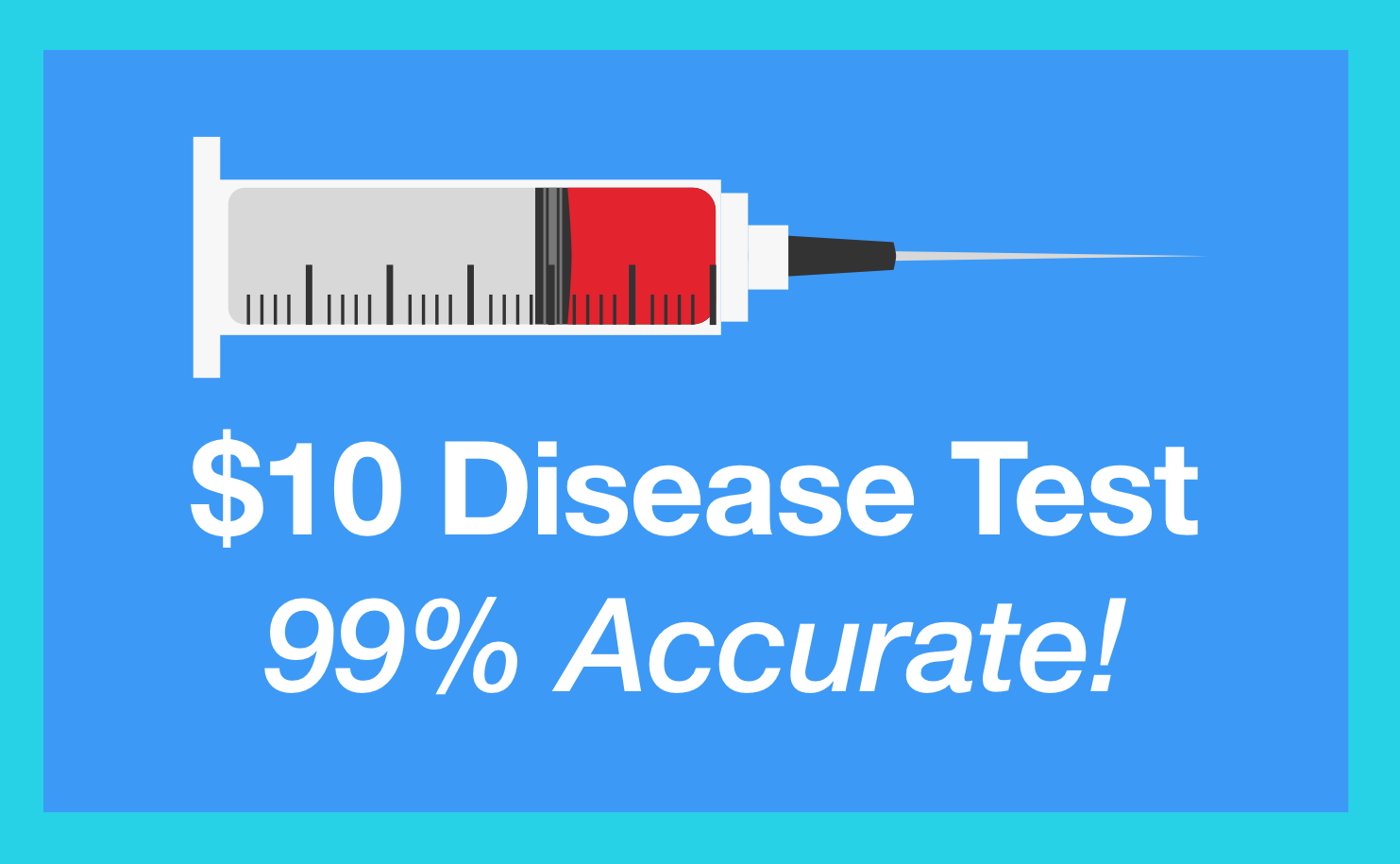A disease test is advertised as being 99% accurate: if you have the disease, you will test positive 99% of the time, and if you don't have the disease, you will test negative 99% of the time.

If 1% of all people have this disease and you test positive, what is the probability that you actually have the disease?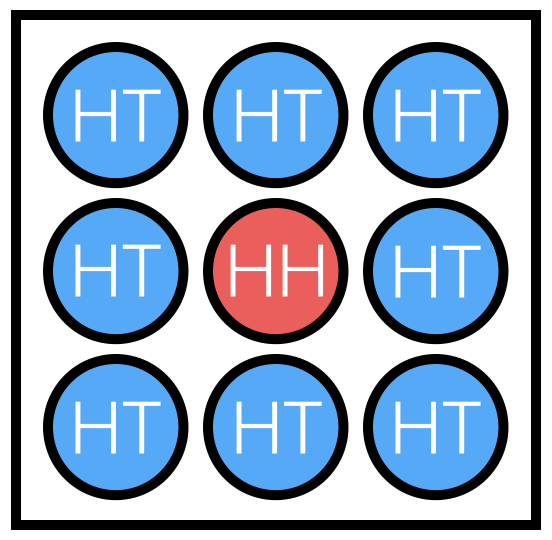Zeb's coin box contains 8 fair, standard coins (heads and tails) and 1 coin which has heads on both sides. He selects a coin randomly and flips it 4 times, getting all heads. If he flips this coin again, what is the probability it will be heads? (The answer value will be from 0 to 1, not as a percentage.)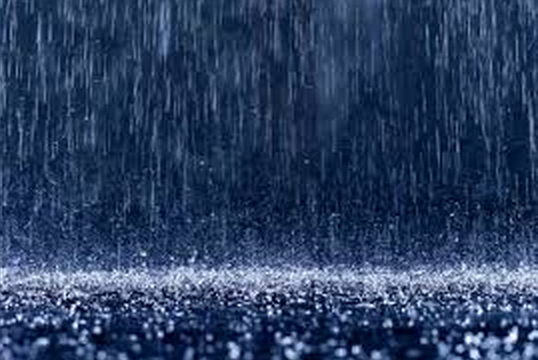In general, the probability that it rains on Saturday is 25%.

If it rains on Saturday, the probability that it rains on Sunday is 50%. If it does not rain on Saturday, the probability that it rains on Sunday is 25%.

Given that it rained on Sunday, what is the probability that it rained on Saturday?

×